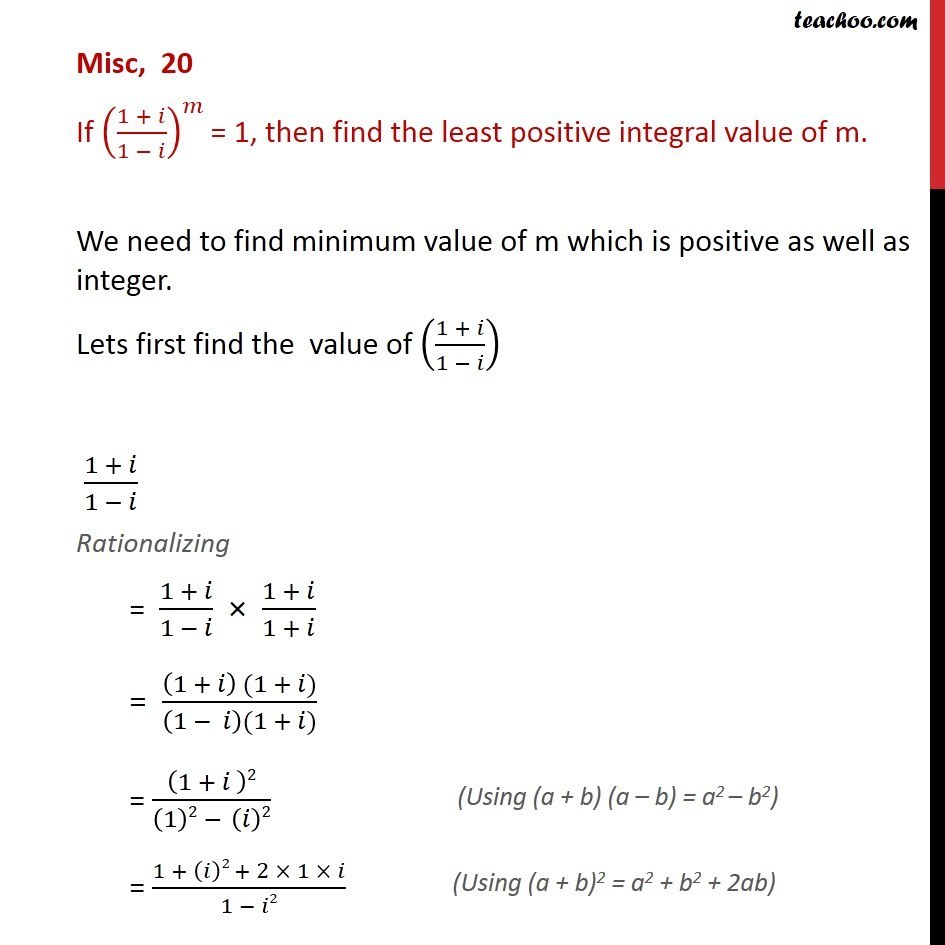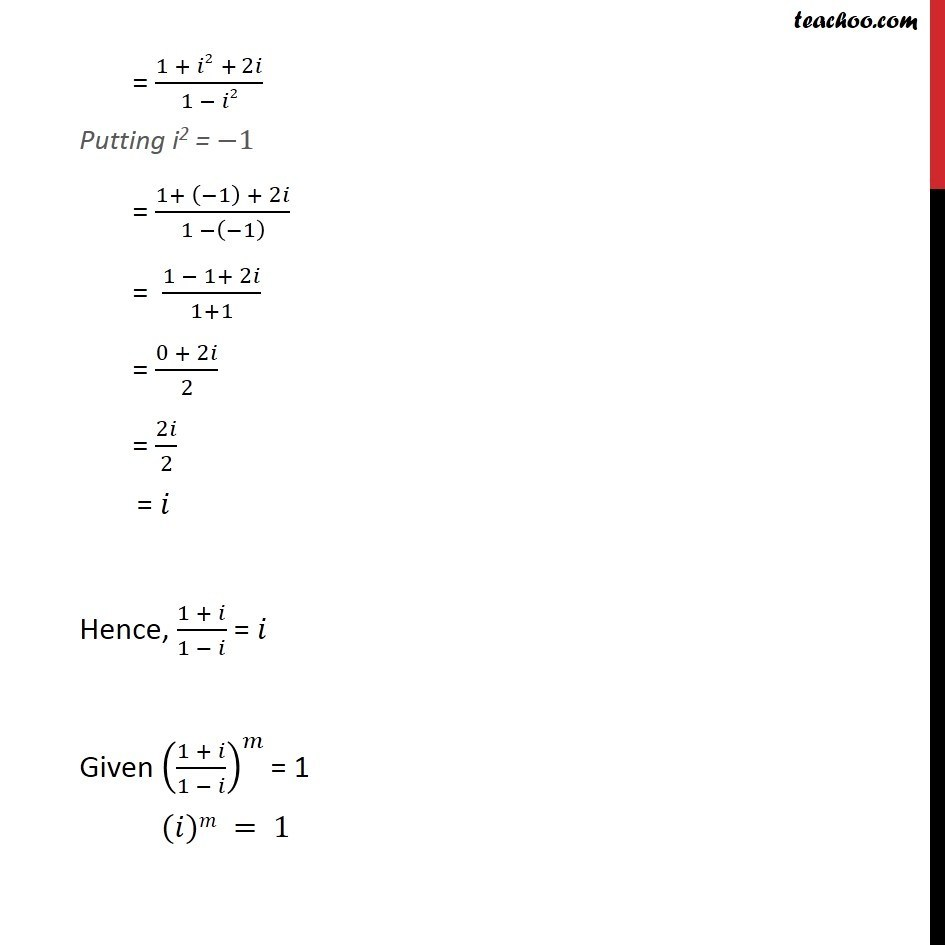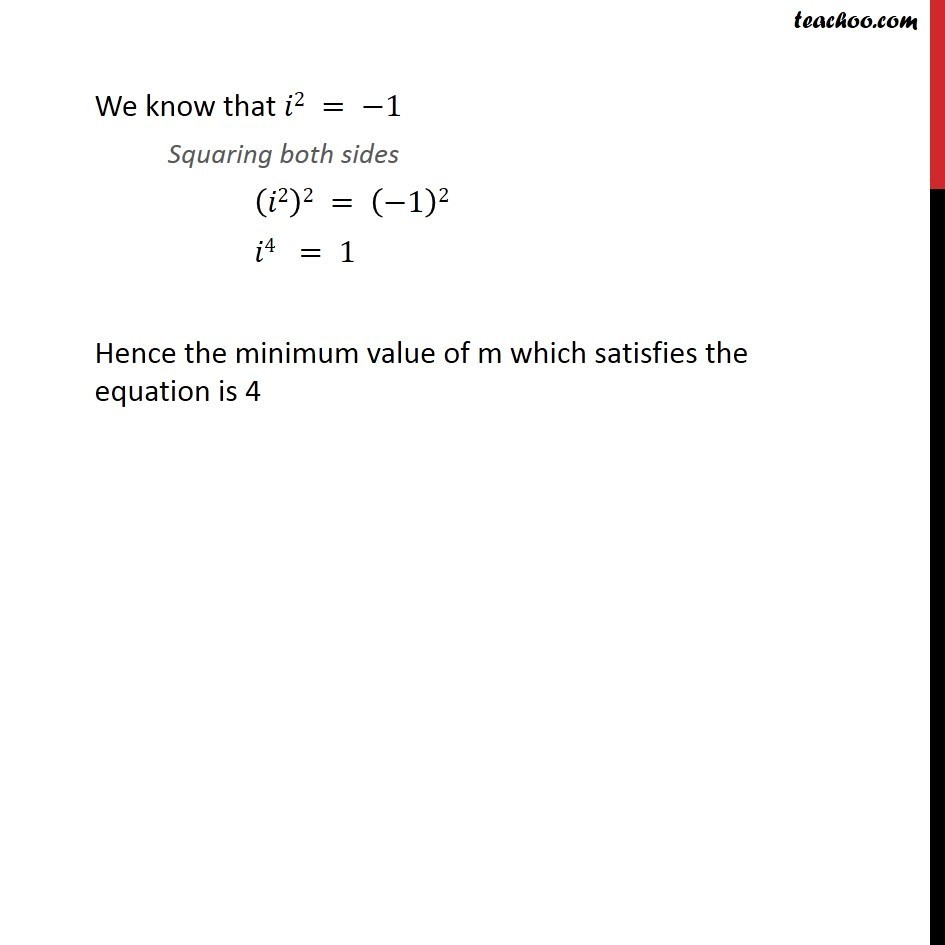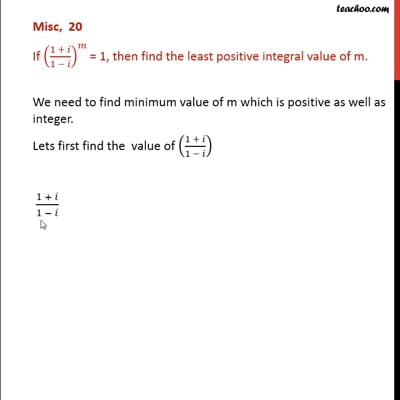Miscellaneous

Chapter 5 Class 11 Complex Numbers
Serial order wiseThis video is only available for Teachoo black users

Solve all your doubts with Teachoo Black (new monthly pack available now!)

### Transcript

Misc, 20 If ((1 + )/(1 ))^ = 1, then find the least positive integral value of m. We need to find minimum value of m which is positive as well as integer. Lets first find the value of ((1 + )/(1 )) (1 + )/(1 ) Rationalizing = (1 + )/(1 ) (1 + )/(1 + ) = ((1 + ) (1 + ))/((1 )(1 + )) = (1 + )2/((1)2 ( )2) = (1 + ( )2 + 2 1 )/(1 2) = (1 + 2 + 2 )/(1 2) Putting i2 = 1 = (1+ ( 1) + 2 )/(1 ( 1) ) = (1 1+ 2 )/(1+1) = (0 + 2 )/2 = 2 /2 = Hence, (1 + )/(1 ) = Given ((1 + )/(1 ))^ = 1 ( ) = 1 We know that 2 = 1 Squaring both sides ( 2)2 = ( 1)2 4 = 1 Hence the minimum value of m which satisfies the equation is 4